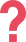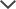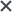## CogAT Test Grade 3 Sample Questions

### CogAT Test Verbal Battery

 CogAT 3rd grade Practice Question-Verbal Analogies The first pair of words are related in a certain way. Choose the word that completes the second pair of words so that they are related in the same way. Peach → fruit : lily →  A) flower B) seed C) tree D) iris E) pit Answer & Explanation ▼ | ▲ The correct answer is A: flower. A peach is a type of fruit like a lily is a type of flower.
 CogAT 3rd grade Practice Question-Verbal Classification Choose the word that best fits with the group. shirt   jacket   socks  A) pockets B) pants C) laundry D) style E) closet Answer & Explanation ▼ | ▲ The correct answer is B: pants. Shirts, jackets, socks and pants are all articles of clothing.
 CogAT 3rd grade Practice Question-Sentence Completion Choose the word that best completes the sentence. Andrew put the shirt back in the clothes dryer as it was still _____. A) damp B) warm C) fresh D) fair E) broken Answer & Explanation ▼ | ▲ The correct answer is A : damp, which means slightly wet. Andrew put the shirt back in the clothes dryer because it was still damp.TestPrep-Online Developer's Tip:

When solving These types of questions (Sentence Completion), one essential tip is to try and identify the type of sentence presented, as it can help you figure out what the missing word should be. Typical sentence types can be Cause and effect, Compare and Contrast, or Definition

### CogAT Test Quantitative Battery​

 CogAT 3rd grade Practice Question-Number Series What is the next number in the series? 0.5     1     1.5     2     2.5     3     3.5     ? A) 4 B) 4.5 C) 5 D) 5.3 E) 45 Answer & Explanation ▼ | ▲ The correct answer is A: 4. In this series, every number is larger than the number before it by one-half (0.5). The pattern is: +0.5, +0.5, +0.5, ... The last number in this series is 3.5, so the next number in the series should be larger than 3.5 by 0.5. 3.5 + 0.5 = 4 Therefore, 4 is the correct answer.
 CogAT 3rd grade Practice Question-Number Puzzles Solve. 26 =  ?  + 9 A) 35 B) 34 C) 16 D) 17 E) 18 Answer & Explanation ▼ | ▲ The correct answer is D: 17. The first thing to notice when solving this type of question is that both sides of the equation must be equal. Since we are trying to solve for the '?', we must get it by itself on one side of the equation. To do this, we must subtract 9 from the right side of the equation, and since both sides of the equation must be equal, we must also subtract 9 from the left side of the equation. 26 - 9 = ? + 9 - 9 On the right side of the equation, we have our '?' by itself. We now have: 26 - 9 = ? To solve for the '?', simply subtract 9 from 26. Be sure to line up the numbers correctly to perform the subtraction: This means that ? = 17.

 CogAT 3rd grade Practice Question-Number analogies Choose the number that completes the third pair so that it demonstrates the same relationship as the first two pairs. [2 → 4]       [17 → 19]       [20 → ?] A) 20 B) 22 C) 25 D) 30 E) 40 Answer & Explanation ▼ | ▲ The answer is B: 22. Look for the pattern in the first series of numbers. The difference between 2 and 4 is +2. The difference between 17 and 19 is also + 2. The next series of numbers should follow the same pattern, meaning that the difference between 20 and ? should be +2 as well: 20 + 2 = 22, meaning that the correct answer will be 22. ? = 22TestPrep-Online Developer's Tip:

When solving Number Analogies questions, you should think of a rule that brings you from the left number to the right one and then use it to find the missing number for the third pair.

If a straightforward rule does not work, try to think of a more complicated connection that involves more than one step. For example, such a relationship can include the addition of a number and then multiplication by another number

### CogAT Test Non-Verbal Battery

CogAT 3rd grade Practice Question-Paper Folding

The paper is being folded and punched with holes. Choose the answer choice that depicts the paper after it is unfolded.• First, the rectangular paper is folded in half lengthwise.
• Then, a small parallelogram is cut out of the folded paper.
• Consequently, the unfolded paper will have two parallelogram cut-outs which eliminates answer choices A and B.
• Since the paper is folded lengthwise, the second parallelogram must mirror horizontally the cut parallelogram which eliminates answer choices D and E.

We are left with the 3rd choice, which is the correct answer.

CogAT 3rd grade Practice Question-Figure Classification

The given shapes have a certain pattern. Choose the answer choice that follows the same pattern.All three arrows are the same size. Choices A, B, C, and D are different in their sizes than the three given arrows. In addition, the arrows are rotating in a clockwise direction, so the missing arrow should be rotated 90 degrees clockwise from the previous image, like in answer E.

Hence, E is the correct answer.

CogAT 3rd grade Practice Question-Figure Matrices

Choose the picture that belongs with the bottom picture in the same way the pictures on top belong together.In the above analogy, the figure on the right is the inverse of the figure on the left. The colors/shading of the outer shape switch with the color/shading of the inner shape. Thus, the answer must be a white triangle with a black arrow inside.TestPrep-Online Developer's Tip:

When solving Figure Matrices questions, you should come up with a rule that brings you from the left figure in the top row to the right one, and then apply this rule in the bottom row to discover the missing figure.

If more than one answer choice fits the rule, look for a more accurate rule. Remember that sometimes there can be more than one rule.

## How to Prepare for the CogAT Grade 3 Test

We recommend that you start preparing two weeks in advance. You can begin by solving our full-length simulation and reading study guides.

When preparing for a test, it is crucial to have as much available information about the test as possible.

The CogAT test is divided into different sections known as batteries: Verbal , Non-verbal , and Quantitative. These batteries feature the following subtopics: Picture/Verbal Analogies, Sentence Completion, Picture/Verbal Classification, Number Analogies, Number Series, Number Puzzles, Figure Matrices, Figure Classification, and Paper Folding.

Each of these subtopics helps develop a different skill. It is essential for your child to see all the different types of questions, their solutions, and the explanations to avoid surprises on test day.

Our practice pack for the CogAT grade 3 contains questions written using the same format as in the CogAT. Each question in our pack has a complete explanation for the answer. Using the explanations, you can double-check your logic or even learn more about the many solving strategies. If you have a challenging questions section, you may approach it after finishing the full-length simulation. In addition to this, you can learn a lot about the different questions using our comprehensive study guides for the various batteries of the test.

## Free CogAT 3rd Grade PDF Practice

View and download a free TestPrep-Online 3rd Grade CogAT Sample Test. This is a printable practice test with various questions and detailed explanations. This would be an excellent starting point for further learning towards the upcoming CogAT test.

## Looking For Related CogAT Free Sample Tests?

-Free CogAT kindergarten sample test

-Free CogAT 1st grade sample test

-Free CogAT 2nd grade sample test

-Free CogAT 4th grade sample test

-Free CogAT 5th grade sample test

-Free CogAT 6th grade sample test

## Need Additional Practice?See What TestPrep-Online Offers

• Level specific, full-length practice tests
• Hundreds of practice questions
• Study guides for children
• Parent manuals

The CogAT(cognitive abilities test) and other trademarks are the property of their respective trademark holders. None of the trademark holders are affiliated with TestPrep-Online or this website.

Reviews

Need HelpNeed Help